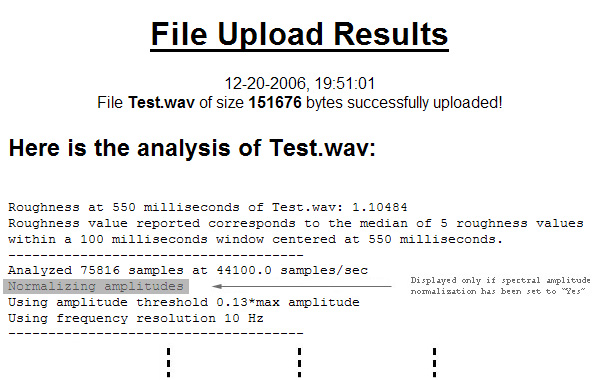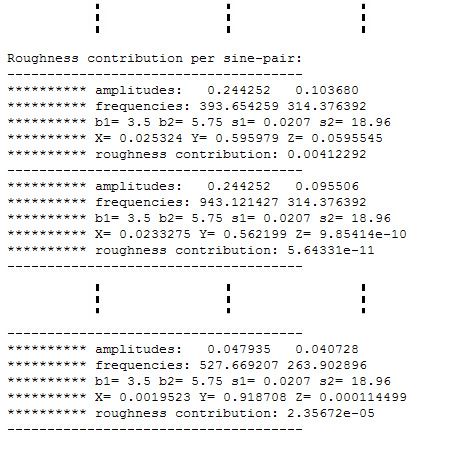Description of the 'Single Roughness' Results Page

Clicking on the 'Single Roughness' button will send your file to the application server for analysis and take you to the 'Results' page. The results will include the roughness value and spectral distribution (frequency and amplitude values by descending amplitude) of the submitted file at the user-defined point in time (default: file's mid-point), and a list of the roughness contributions pair sine-pair within the signal's spectrum. To return from the 'Results' to the 'SRA' page, use the browser's 'Back' button or hit the 'Backspace' key on the keyboard.

The analysis window length will be determined by the frequency resolution setting (~320ms for 10Hz and ~160ms for 20Hz) and be centered at the user-defined point in time. If the 'milliseconds' field is left blank, the analysis window will be centered at the file's mid-point. The earliest (latest) point within a file for which a roughness value can be calculated is 158ms (for 10Hz frequency resolution; 79ms for 20Hz frequency resolution) from the beginning (end) of the submitted file.

The degree of precision of the spectral analysis (in terms of time, frequency, and amplitude estimates) is much finer than the one required for roughness calculation (read about the spectral analysis method). It captures fast, fine spectral time-variance that does not necessarily correspond to perceivable roughness variations.
To reduce this effect, the (a) roughness value, (b) time value, and (c) spectrum returned from analysis at a requested time, reflect
a) the median of 5 roughness values calculated from 5 equally-spaced spectra within an 100ms window (i.e. at -50ms, -25ms, 0ms, +25ms, and +50ms), centered at the time requested,
b) the time corresponding to the reported roughness value (possibly different from the requested time), and
c) the spectrum used to calculate the reported roughness value.

The sections below outline the segments of the 'Single Roughness' Results page and include:
a) the roughness and time values returned from the analysis and a report of the analysis parameters,
b) the spectral distribution (by descending amplitude) corresponding to the reported roughness, and
c) the roughness contribution per sine-pair in the submitted file's spectrum, along with the relevant calculations.

ROUGHNESS VALUE AT THE REQUESTED TIME - ANALYSIS PARAMETERSFigure 1: The results display the roughness value calculated at the time requested (e.g. 550ms). If the time field had been left blank, the roughness report would read: "Roughness at the middle of ...". A statement on the roughness calculation's smoothening function is followed by an outline of the file and analysis parameters.

SPECTRAL DISTRIBUTION AT THE REQUESTED TIMEFigure 2: The spectral distribution consists of the frequency and amplitude values of up to 50 'peaks' (components) with amplitudes > the amplitude determined by the amplitude threshold value, listed by descending amplitude. Regardless of the decimal digits displayed, frequency and amplitude values are estimated at 32-bit, floating-point precision. Note that the time indicated in this portion of the results (e.g. 500ms, Figure 2) will not necessarily be the same as the user-defined time, reported in the top portion of the results (e.g. 550ms, Figure 1). As already described, the roughness value reported at any given time corresponds to the median of 5 roughness values calculated from 5 equally-spaced spectra within an 100ms window (i.e. at -50ms, -25ms, 0ms, +25ms, and +50ms), centered at the time requested. See the top of this page for more.

ROUGHNESS CONTRIBUTION PER SINE-PAIRFigure 3: The roughness contribution of each sine-pair includes the parameter values of the model and the values for each of the 3 model terms (see the model description). The total roughness, reported at the top of the 'Results' page equals the sum of all sine-pair contributions, scaled-up by a factor of 100, selected to produce manageable roughness values.